# 决策树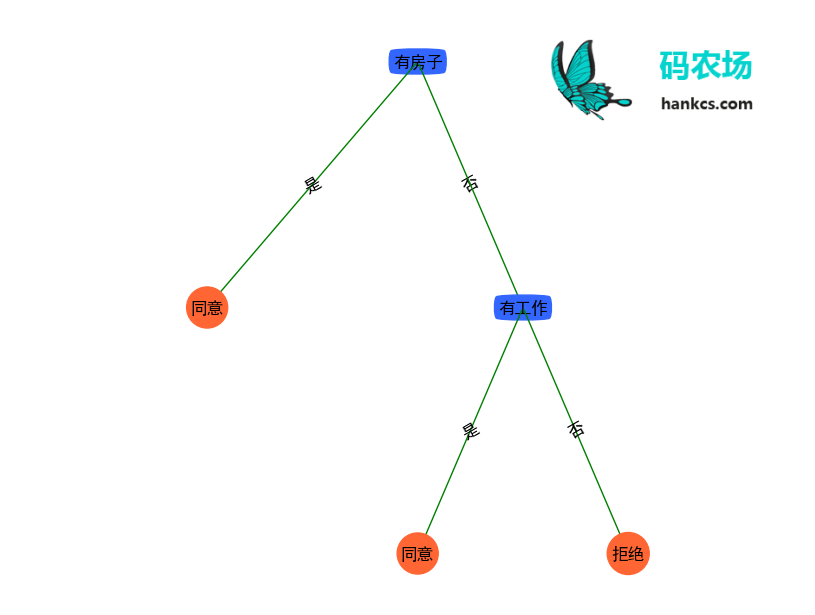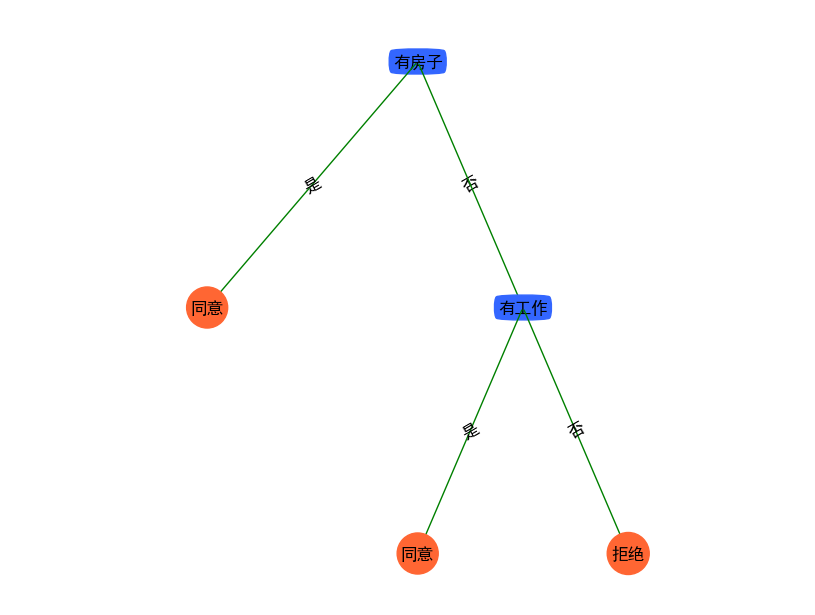“何以别离久，何以少团栾。”

## 决策树模型与学习

### 决策树与条件概率分布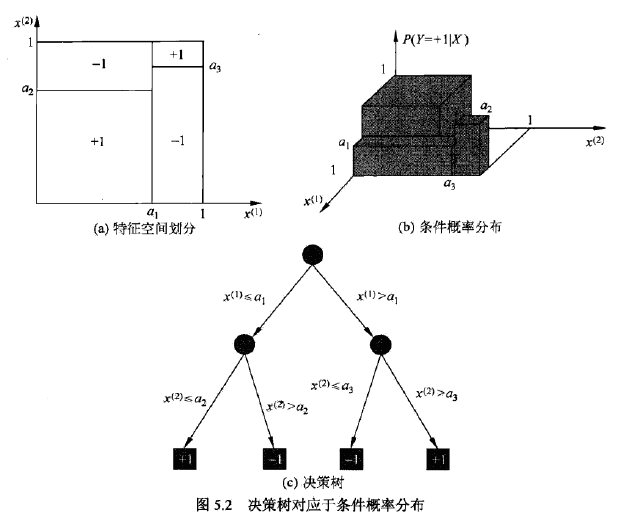### 决策树学习

def majorityCnt(classList):
"""

:param classList: 类列表
:return: 出现次数最多的类名称
"""
classCount = {}  # 这是一个字典
for vote in classList:
if vote not in classCount.keys(): classCount[vote] = 0
classCount[vote] += 1
sortedClassCount = sorted(classCount.iteritems(), key=operator.itemgetter(1), reverse=True)
return sortedClassCount

def createTree(dataSet, labels, chooseBestFeatureToSplitFunc=chooseBestFeatureToSplitByID3):
"""

:param dataSet:数据集
:param labels:数据集每一维的名称
:return:决策树
"""
classList = [example[-1] for example in dataSet]  # 类别列表
if classList.count(classList) == len(classList):
return classList  # 当类别完全相同则停止继续划分
if len(dataSet) == 1:  # 当只有一个特征的时候，遍历完所有实例返回出现次数最多的类别
return majorityCnt(classList)
bestFeat = chooseBestFeatureToSplitFunc(dataSet)
bestFeatLabel = labels[bestFeat]
myTree = {bestFeatLabel: {}}
del (labels[bestFeat])
featValues = [example[bestFeat] for example in dataSet]
uniqueVals = set(featValues)
for value in uniqueVals:
subLabels = labels[:]  # 复制操作
myTree[bestFeatLabel][value] = createTree(splitDataSet(dataSet, bestFeat, value), subLabels)
return myTree

## 特征选择

### 特征选择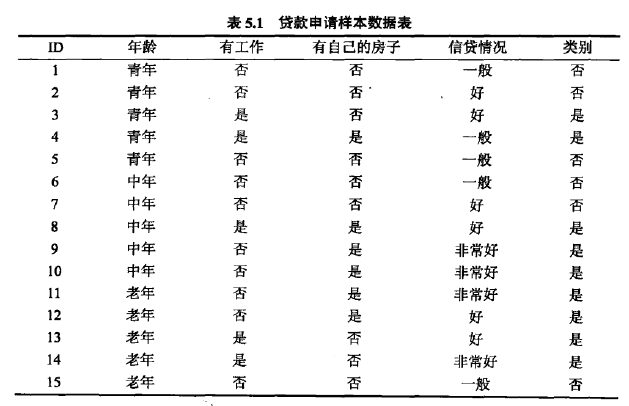def createDataSet():
"""

:return:
"""
dataSet = [[u'青年', u'否', u'否', u'一般', u'拒绝'],
[u'青年', u'否', u'否', u'好', u'拒绝'],
[u'青年', u'是', u'否', u'好', u'同意'],
[u'青年', u'是', u'是', u'一般', u'同意'],
[u'青年', u'否', u'否', u'一般', u'拒绝'],
[u'中年', u'否', u'否', u'一般', u'拒绝'],
[u'中年', u'否', u'否', u'好', u'拒绝'],
[u'中年', u'是', u'是', u'好', u'同意'],
[u'中年', u'否', u'是', u'非常好', u'同意'],
[u'中年', u'否', u'是', u'非常好', u'同意'],
[u'老年', u'否', u'是', u'非常好', u'同意'],
[u'老年', u'否', u'是', u'好', u'同意'],
[u'老年', u'是', u'否', u'好', u'同意'],
[u'老年', u'是', u'否', u'非常好', u'同意'],
[u'老年', u'否', u'否', u'一般', u'拒绝'],
]
labels = [u'年龄', u'有工作', u'有房子', u'信贷情况']
# 返回数据集和每个维度的名称
return dataSet, labels

def splitDataSet(dataSet, axis, value):
"""

:param dataSet: 待划分的数据集
:param axis: 划分数据集的特征的维度
:param value: 特征的值
:return: 符合该特征的所有实例（并且自动移除掉这维特征）
"""
retDataSet = []
for featVec in dataSet:
if featVec[axis] == value:
reducedFeatVec = featVec[:axis]  # 删掉这一维特征
reducedFeatVec.extend(featVec[axis + 1:])
retDataSet.append(reducedFeatVec)
return retDataSet

### 信息增益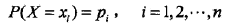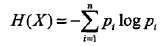def calcShannonEnt(dataSet):
"""

:param dataSet:
:return:
"""
numEntries = len(dataSet)  # 实例的个数
labelCounts = {}
for featVec in dataSet:  # 遍历每个实例，统计标签的频次
currentLabel = featVec[-1]
if currentLabel not in labelCounts.keys(): labelCounts[currentLabel] = 0
labelCounts[currentLabel] += 1
shannonEnt = 0.0
for key in labelCounts:
prob = float(labelCounts[key]) / numEntries
shannonEnt -= prob * log(prob, 2)  # log base 2
return shannonEnt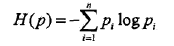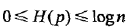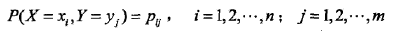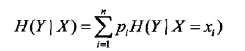Python实现条件熵的计算

def calcConditionalEntropy(dataSet, i, featList, uniqueVals):
'''
计算X_i给定的条件下，Y的条件熵
:param dataSet:数据集
:param i:维度i
:param featList: 数据集特征列表
:param uniqueVals: 数据集特征集合
:return:条件熵
'''
ce = 0.0
for value in uniqueVals:
subDataSet = splitDataSet(dataSet, i, value)
prob = len(subDataSet) / float(len(dataSet))  # 极大似然估计概率
ce += prob * calcShannonEnt(subDataSet)  # ∑pH(Y|X=xi) 条件熵的计算
return ce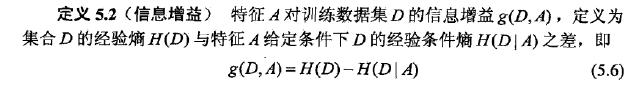def calcInformationGain(dataSet, baseEntropy, i):
"""
计算信息增益
:param dataSet:数据集
:param baseEntropy:数据集中Y的信息熵
:param i: 特征维度i
:return: 特征i对数据集的信息增益g(dataSet|X_i)
"""
featList = [example[i] for example in dataSet]  # 第i维特征列表
uniqueVals = set(featList)  # 转换成集合
newEntropy = calcConditionalEntropy(dataSet, i, featList, uniqueVals)
infoGain = baseEntropy - newEntropy  # 信息增益，就是熵的减少，也就是不确定性的减少
return infoGain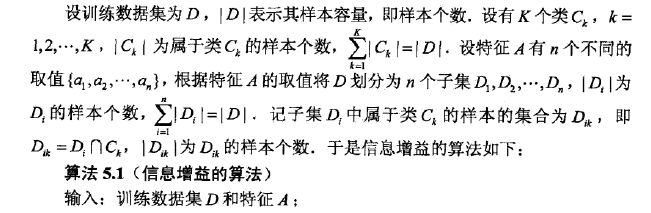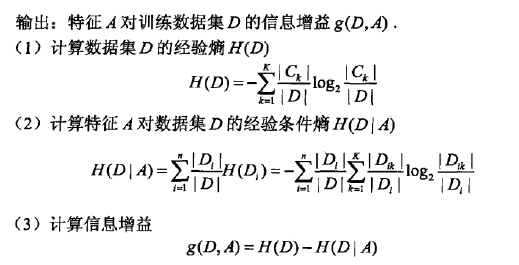### 信息增益比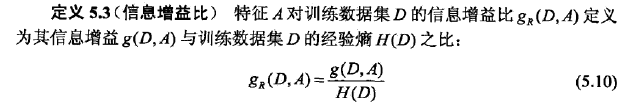$$H_A(D) = -\sum\limits_{i=1}^{n}\frac{|D_i|}{|D|}log_2\frac{|D_i|}{|D|}$$

Python代码：

def calcInformationGainRate(dataSet, baseEntropy, i):
"""
计算信息增益比
:param dataSet:数据集
:param baseEntropy:数据集中Y的信息熵
:param i: 特征维度i
:return: 特征i对数据集的信息增益g(dataSet|X_i)
"""
return calcInformationGain(dataSet, baseEntropy, i) / baseEntropy

## 决策树的生成

### Python实现

ID3特征选择算法的Python实现：

def chooseBestFeatureToSplitByID3(dataSet):
"""

:param dataSet:
:return:
"""
numFeatures = len(dataSet) - 1  # 最后一列是分类
baseEntropy = calcShannonEnt(dataSet)
bestInfoGain = 0.0
bestFeature = -1
for i in range(numFeatures):  # 遍历所有维度特征
infoGain = calcInformationGain(dataSet, baseEntropy, i)
if (infoGain > bestInfoGain):  # 选择最大的信息增益
bestInfoGain = infoGain
bestFeature = i
return bestFeature  # 返回最佳特征对应的维度

# -*- coding:utf-8 -*-
# Filename: testTree.py
# Author：hankcs
# Date: 2014-04-19 下午9:19

###########中文支持################
import sys
from tree import *

sys.setdefaultencoding('utf-8')
from pylab import *

mpl.rcParams['font.sans-serif'] = ['SimHei']  # 指定默认字体
mpl.rcParams['axes.unicode_minus'] = False  # 解决保存图像时负号'-'显示为方块的问题
##################################

# 测试决策树的构建
myDat, labels = createDataSet()
myTree = createTree(myDat, labels)
# 绘制决策树
import treePlotter
treePlotter.createPlot(myTree)

### 可视化# -*- coding:utf-8 -*-
# Filename: treePlotter.py
# Author：hankcs
# Date: 2015/2/9 21:24
import matplotlib.pyplot as plt

# 定义文本框和箭头格式
decisionNode = dict(boxstyle="round4", color='#3366FF')  #定义判断结点形态
leafNode = dict(boxstyle="circle", color='#FF6633')  #定义叶结点形态
arrow_args = dict(arrowstyle="<-", color='g')  #定义箭头

#绘制带箭头的注释
def plotNode(nodeTxt, centerPt, parentPt, nodeType):
createPlot.ax1.annotate(nodeTxt, xy=parentPt, xycoords='axes fraction',
xytext=centerPt, textcoords='axes fraction',
va="center", ha="center", bbox=nodeType, arrowprops=arrow_args)

#计算叶结点数
def getNumLeafs(myTree):
numLeafs = 0
firstStr = myTree.keys()
secondDict = myTree[firstStr]
for key in secondDict.keys():
if type(secondDict[key]).__name__ == 'dict':
numLeafs += getNumLeafs(secondDict[key])
else:
numLeafs += 1
return numLeafs

#计算树的层数
def getTreeDepth(myTree):
maxDepth = 0
firstStr = myTree.keys()
secondDict = myTree[firstStr]
for key in secondDict.keys():
if type(secondDict[key]).__name__ == 'dict':
thisDepth = 1 + getTreeDepth(secondDict[key])
else:
thisDepth = 1
if thisDepth > maxDepth:
maxDepth = thisDepth
return maxDepth

#在父子结点间填充文本信息
def plotMidText(cntrPt, parentPt, txtString):
xMid = (parentPt - cntrPt) / 2.0 + cntrPt
yMid = (parentPt - cntrPt) / 2.0 + cntrPt
createPlot.ax1.text(xMid, yMid, txtString, va="center", ha="center", rotation=30)

def plotTree(myTree, parentPt, nodeTxt):
numLeafs = getNumLeafs(myTree)
depth = getTreeDepth(myTree)
firstStr = myTree.keys()
cntrPt = (plotTree.xOff + (1.0 + float(numLeafs)) / 2.0 / plotTree.totalW, plotTree.yOff)
plotMidText(cntrPt, parentPt, nodeTxt)  #在父子结点间填充文本信息
plotNode(firstStr, cntrPt, parentPt, decisionNode)  #绘制带箭头的注释
secondDict = myTree[firstStr]
plotTree.yOff = plotTree.yOff - 1.0 / plotTree.totalD
for key in secondDict.keys():
if type(secondDict[key]).__name__ == 'dict':
plotTree(secondDict[key], cntrPt, str(key))
else:
plotTree.xOff = plotTree.xOff + 1.0 / plotTree.totalW
plotNode(secondDict[key], (plotTree.xOff, plotTree.yOff), cntrPt, leafNode)
plotMidText((plotTree.xOff, plotTree.yOff), cntrPt, str(key))
plotTree.yOff = plotTree.yOff + 1.0 / plotTree.totalD

def createPlot(inTree):
fig = plt.figure(1, facecolor='white')
fig.clf()
axprops = dict(xticks=[], yticks=[])
createPlot.ax1 = plt.subplot(111, frameon=False, **axprops)
plotTree.totalW = float(getNumLeafs(inTree))
plotTree.totalD = float(getTreeDepth(inTree))
plotTree.xOff = -0.5 / plotTree.totalW;
plotTree.yOff = 1.0;
plotTree(inTree, (0.5, 1.0), '')
plt.show()

### C4.5的生成算法

C4.5算法与ID3相似，但是在选择的时候使用的是信息增益比，形式化地描述如下：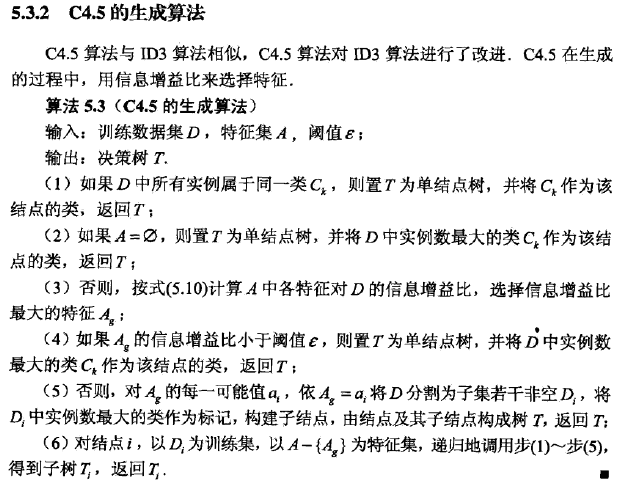### Python实现

def chooseBestFeatureToSplitByC45(dataSet):
"""

:param dataSet:
:return:
"""
numFeatures = len(dataSet) - 1  # 最后一列是分类
baseEntropy = calcShannonEnt(dataSet)
bestInfoGainRate = 0.0
bestFeature = -1
for i in range(numFeatures):  # 遍历所有维度特征
infoGainRate = calcInformationGainRate(dataSet, baseEntropy, i)
if (infoGainRate > bestInfoGainRate):  # 选择最大的信息增益
bestInfoGainRate = infoGainRate
bestFeature = i
return bestFeature  # 返回最佳特征对应的维度

myTree = createTree(myDat, labels, chooseBestFeatureToSplitByC45)

### 可视化## 决策树的剪枝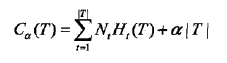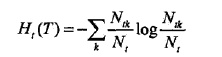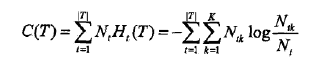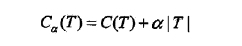C(T)表示模型对训练数据的预测误差，即模型与训练数据的拟合程度，|T|表示模型复杂度，参数α≥0控制两者之间的影响，α越大，模型越简单，α=0表示不考虑复杂度。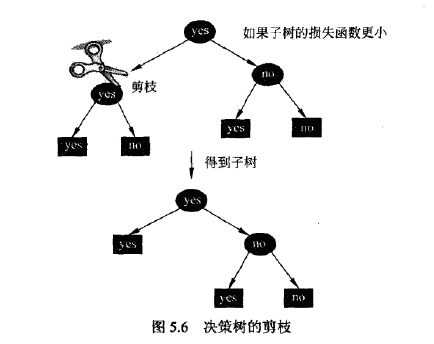## CART算法

### CART生成

#### 1、回归树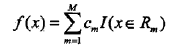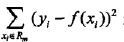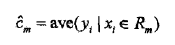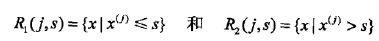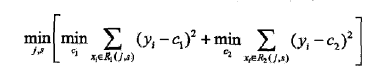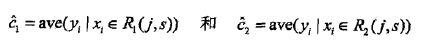#### 2、分类树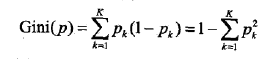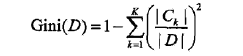Ck是属于第k类的样本子集，K是类的个数。Gini(D)反应的是D的不确定性（与熵类似），分区的目标就是降低不确定性。

D根据特征A是否取某一个可能值a而分为D1和D2两部分：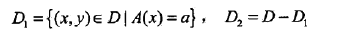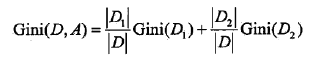### CART剪枝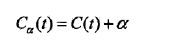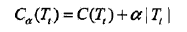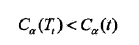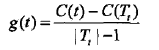### 评论 8

1. #5

求问，为什么没实现这里的剪枝算法呢？我不是很懂书中算法5.4中的经验熵说法，这里说“设决策树T的叶节点有|T|个，t是某个叶节点，t有Nt个样本点，其中归入k类的样本点有Ntk个”，但是决策树中的所有叶节点的Nt个样本点，不都是一类的吗（就是标签都是一样的），也就是Ntk = Nt，那这样所有叶节点经验熵Ht(T)不都是0了吗？这里该如何理解呢？

lau5年前 (2018-12-18)回复
2. #4

大神你好，在你的calcInformationGainRate函数最后一行，你的baseEntropy是H(D)而不是HA(D)吧。

一个渣渣6年前 (2017-07-25)回复
3. #3

博主你好。有个小问题，计算信息增益比的时候，分母应该是HA(D)，而不应该是H(D)吧。如有不对，还请见谅。

苏大天行健7年前 (2017-07-10)回复
• 感谢指正，你是对的，分母应该是数据集中特征A的split information value $H_A(D) = -\sum\limits_{i=1}^{n}\frac{|D_i|}{|D|}log_2\frac{|D_i|}{|D|}$

hankcs7年前 (2017-07-10)回复
4. #2

说错了，应该是“对每一个特征的信息增益来说……”

游行至8年前 (2015-12-25)回复
• ID3是通过计算信息增益来选择特征，C4.5是通过计算信息增益比来选择。

燕鹏_7年前 (2016-07-28)回复
5. #1

我觉得ID3算法和C4.5算法没区别啊，因为对每一个特征的信息分类来说，H(D)都是一样的啊，只需要比较H(D|A)就可以了啊。

游行至8年前 (2015-12-25)回复
• 我也想问信息增益公式中为什么不去掉 H(D) 呢？

sbzcyg7年前 (2017-07-10)回复